# Vector Algebra

## What is vector

• A quantity  that has magnitude as well as direction is called vector.From a geometric point of view, a vector can be defined as a line segment having a specific direction and a specific length
• It is denoted  by the letter bold letter a  or it can be denoted as a
• Magnitude of a vector a is denoted by |a| or a.It is a positive quantity

## Difference between Scalar and Vector

Physical quantities may be divided into two categories
(1) Scalars are physical quantities that only have magnitude for example mass, length, time, temperature etc.
(2)Vectors are physical quantities having both magnitude and direction for example velocity, force, electric field, torque etc. It can be represented by an arrow in space.

## Type Of Vectors

(i) Zero or Null Vector :A vector whose initial and terminal points are coincident is called zero or null vector
(ii) Unit Vector :A vector whose magnitude is unity is called a unit vector which is denoted by n^
(iii) Free Vectors: If the initial point of a vector is not specified, then it is said to be a free vector.
(iv) Negative of a Vector A vector having the same magnitude as that of a given vector a and the direction opposite to that of a is called the negative of a and it is denoted by —a.
(v) Like and Unlike Vectors Vectors are said to be like when they have the same direction and unlike when they have opposite direction.
(vi) Collinear or Parallel Vectors Vectors having the same or parallel supports are called collinear vectors.
(vii) Coinitial Vectors Vectors having same initial point are called coinitial vectors.
(viii) Coplanar Vectors A system of vectors is said to be coplanar, if their supports are parallel to the same plane. Otherwise they are called non-coplanar vectors
(iX) Equal vectors: Two vectors a and b are said to be equal written as a = b, if they have  same magnitude and same direction regardless of the there initial point

Let a and b be any two vectors. From the terminal point of a, vector b is drawn. Then, the vector from the initial point  of a to the terminal point B of b is called the sum of vectors a and b and is denoted by a + b. This is called the triangle law of addition of vectors
c= a + b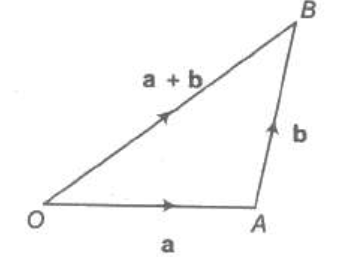(1) Vector addition is commutative i.e. A+B=B+A
(2) Vector addition is associative i.e. A+B+C=(A+B)+C=A+(B+C)=(A+C)+B
(3)  A +0= A
4) A+ (-A)=0

## Subtraction of vectors

Let a and b be any two vectors.
Now a-b = a+ (-b)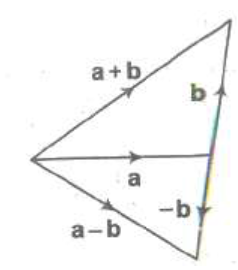So we will first reverse the direction of vector b and then follow the vector addition process
From the terminal point of a, vector -b is drawn. Then, the vector from the initial point  of a to the terminal point B of -b is called the sum of vectors a and -b and is denoted by a - b.

Another method to find substraction of vectors would be
Let draw vector a and vector b from the same initial point. And then draw the line from end point of vector b to vector a.This will  give a-b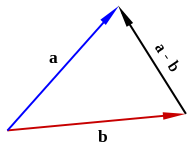## Scalar multiplication of vectors

When we multiply any vector A with any scalar quantity 'n' then it's direction remains unchanged and magnitude gets multiplied by 'n'. Thus, n(A) = nA
Scalar multiplication of vectors is distributive i.e.,
n(A + B) = nA +nB

Important Properties
(i) |k a| = |k| |a|
(ii) k O = O
(iii) m (-a) = – ma = – (m a)
(iv) (-m) (-a) = m a
(v) m (n a) = mn a = n(m a)
(vi) (m + n)a = m a+ n a
(vii) m (a+b) = m a + m b

## Components of the vector

We can  represent any vector in rectangular components form. Let us assume an xyz coordinate plane and unit vector i,j and k are defined across x,y,z respectively
Then we can represent any vector in the components forms like
r= xi+yj+ zk
Important take aways
• x,y and z are scalar components of vector r
• xi,yj,zk are called the vector components
• x,y,z are termed as rectagular components
• Length of vector or magnitude of the vector is defined as
•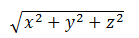• x,y,z are called the direction ratio of vector r
• In case it is given l,m,n are direction cosines of a vector then
li+mj+nk=(cosθx) i +(cosθy) j+(cosθz) k    is the unit vector in the direction of the vector and θx,θy,θz    are the angles which the vector makes with x,y and z axis
Addition,substraction and multiplication,equality in component form can be expressed
In component form addition of two vectors is
C=A+B
C = (Ax+ Bx)i + (Ay+ By)j + (Az+ Bz)k
Where, A = (Ax, Ay, Az) and B = (Bx, By, Bz)
Thus in component form resultant vector C becomes,
Cx = Ax+ Bx : Cy = Ay+ By : Cz = Az+ Bz
In component form  substraction of two vectors is
D=A -B
D = (Ax- Bx)i + (Ay- By)j + (Az- Bz)k
where, A = (Ax, Ay, Az) and B = (Bx, By, Bz)
Thus in component form resultant vector D becomes,
Dx = Ax - Bx : Dy = Ay- By : Dz = Az- Bz

Equality of vector in components form
A=B
Axi +Ayj+Azk= Bxi+Byj+Bzk
Ax=Bx
Ay=By
Az=Bz
Multiplication of scalar by vector in components form
A=kB
=K(Bxi+Byj+Bzk)
=(kBx)i + (kBy)j+ (kBz)k
Position vector
In the coordinate system, the line joining the origin O to the point P in the system is called the position vector of Point P
OP= xi+yj+zk
Vector joining two points in the Coordinate system
Let Point P and Q are there
Postion Vector of Point P
OP= x1 i+y1 j+z1k
Postion Vector of Point Q
OQ= x2 i+y2 j+z2k
Then Vector PQ is defined as
PQ=OQ-OP
=(x2 -x1) i +(y2 -y1) j +(z2 -z1) k
Collinear Vectors
Vectors a and b are collinear, if a = λb, for some non-zero scalar λ.
Collinear Points
Let A, B, C be any three points.
Points A, B, C are collinear <=> AB, BC are collinear vectors.
<=> AB = kBC for some non-zero scalar k
Section Formula
Let A and B be two points with position vectors  a and b, respectively
(i) Let P be a point dividing AB internally in the ratio m : n. Then,
r = m b + n a / m + n
where r is the position vector of point P
(ii) The position vector of the mid-point of a and b is a + b / 2.
(iii) Let P be a point dividing AB externally in the ratio m : n. Then,
r = m b + n a / m + n
Multiplication of two vectors Multiplication of Vectors can be done in two ways
Dot Product
Cross Product

## Dot product of vectors

• Dot product of two vectors A and B is defined as the product of the magnitudes of vectors A and B and the cosine of the angle between them when both te vectors are placed tail to tail. Dot product is represented as A.B thus,
A.B = |A| |B| cosθ
where θ is the angle between two vectors and  0 =< θ <= 180
• Result of dot product of two vectors is a scalar quantity.
•  Dot product is commutative : A.B = B.A
•  Dot product is distributive : A . (B+C) = A.B + A.C also A.A = |A|2
•  In component form dot product of two vectors
A.B = (Axi + Ayj + Azk) . (Bxi + Byj + Bzk) = AxBx + AyBy + AzBz.
Thus for calculating the dot product of two vectors, first multiply like components, and then add.
•  For two mutually perpendicular vectors A.B = 0
•  Scalar product of two equal vectors is A.A=|A|2
• If either a or b is the null vector, then scalar product of the vector is zero.
• If a and b are two unit vectors, then a * b = cos θ
If i , j and k are mutually perpendicular unit vectors i , j and k, then
i * i = j * j = k * k =1
and i * j = j * k = k * i = 0
Angle between Two Vectors If θ is angle between two non-zero vectors, a, b, then we have
a * b = |a| |b| cos θ
cos θ = a * b / |a| |b|

## Cross product of vectors

•  Cross product or vector product of two vectors A and B is defined as
A x B = |A| |B| sinθ n ?
where n ? is the unit vector pointing in the direction perpendicular to the plane of both A and B. Result of vector product is also a vector quantity.
•  Cross product is distributive i.e., A x (B + C) = (A x B) + (A x C) but not commutative and
•  The cross product of two parallel vectors is zero.
•  Component of any vector is the projection of that vector along the three coordinate axes X, Y, Z.
A x B = (Axi + Ayj + Azk) x (Bxi + Byj + Bzk)
= (AyBz - AzBy)i + (AzBx - AxBz)j + ( AxBy - AyBx)k.
•  Cross product of two vectors is itself a vector.
•  To calculate the cross product, form the determinant whose first row is x, y, z, whose second row is A (in component form), and whose third row is B.

## Triple product

Vector product of two vectors can be made to undergo dot or cross product with any third vector.
(a) Scalar tripple product:-
• For three vectors A, B, and C, their scalar triple product is defined as A . (B x C) = B . (C x A) = C . (A x B) obtained in cyclic permutation.
• Switching the two vectors in the cross product negates the triple product, i.e.:   C . (A x B) =-C . (B x A)
• If A = (Ax, Ay, Az) , B = (Bx, By, Bz) , and C = (Cx, Cy, Cz) then, A . (B x C) is the volume of a parallelepiped having A, B, and C as edges and can easily obtained by finding the determinant of the 3 x 3 matrix formed by AB, and C.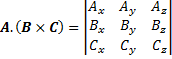• If the scalar triple product is equal to zero, then the three vectors a, b, and c are coplanar, since the "parallelepiped" defined by them would be flat and have no volume.
(b) Vector Triple Product:-
• For vectors A, B, and C, we define the vector tiple product as A x (B x C) = B(A . C) - C(A - B)
• Note that (A × B) ×C ≠ A× (B × C)

## Related Topics

Go back to Class 12 Main Page using below links